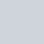# Geometric Mean definiton, formula and applications

The Geometric mean is a special types of average where we calculate the root of the product of a value of a set of observations. It is usually used to define various types of growth rates such as population growth, interest rates etc.

### Formula of geometric mean

By definition, the formula is as below,

G.M =Where, G.M=geometric mean

n=number of observations

### Mathematical example

What is the geometric mean of 2, 3, and 6?
First, multiply the numbers together and then take the cubed root (because there are three numbers) = (2*3*6)1/3 = 3.30

Note: The power of (1/3) is the same as the cubed root 3√. To convert a nth root to this notation, just change the denominator in the fraction to whatever “n” you have. So,

• 5th root = to the (1/5) power
• 12th root = to the (1/12) power
• 99th root = to the (1/99) power.…….

### The situation where we use geometric mean

The geometric mean is the most appropriate measure of location when,

It is appropriate for averaging the ratios of change, for average of proportions, etc.

There are some merits as following,

• Geometric mean is least affected by extreme values.
• It is based on all observations of the set.
• It is suitable for further algebraic treatment.

There are some demerits as following,

• Geometric mean calculation is somewhat complicated.
• It cannot be calculated if any of the values in the set is zero.
• If any one or more values are negative, either it will not be calculable, or an absurd value will be obtained.

### 4 thoughts on “Geometric Mean definiton, formula and applications”

1.Ginger

blog, I have read all that, so now me also commenting at this
place.

2.situs slot

With havin so much content and articles do you ever run into any issues
of plagorism or copyright infringement? My blog has a lot of completely unique content I’ve either written myself or outsourced but it appears a lot
of it is popping it up all over the internet without my permission. Do you know any ways to help prevent content from being
stolen? I’d certainly appreciate it.

3.gsa link

It will automatically pull verified URLS from SER and submit them
to statistic/whois web pages.

Look at my web page: gsa link

4.pragmatic

This article gives clear idea in favor of the new viewers of
blogging, that truly how to do running a blog.